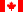Canadian Version Return to Calculator : Help - Contents

### Significant Figures

The precision of a calculated value cannot exceed the precision of any of the inputted variables. Therefore, it must be rounded to the least number of significant figures of either No or N. To increase the precision of the calculated value, increase the number of significant figures in the initial values that you enter by using scientific notation. This calculator conforms to typical "calculator-style" scientific notation, where E is equivalent to x10^.

e.g.     500 = 5x102 = 5E2       0.05 = 5x10-2 = 5E-2

Note that in this example, the value 500 has only one significant figure, but depending on the level of uncertainty, it could actually have 1, 2, 3, or even an infinite number of significant figures if it were treated as an exact number. Exact numbers are sometimes called counting numbers; for example, if there are 3 people in the room, you can say that there are 3.000000000... people in the room. However if you have 3 inches of string, you can't say for certain that you have 3.000000000... inches of string unless your measurement of the string has that level of precision.

For this calculator, values of Time (t) which are entered as whole numbers (no decimal) are treated as exact numbers. Initial and final activities entered as whole numbers are not.

Significant Figures and Scientific Notation

 Example ScientificNotation Significant Figures 37000 3.7E4 2 37000. 3.7000E4 5 37000.00 3.700000E4 7 0.0037 3.7E-3 2 0.0037000 3.7000E-3 5

The following table illustrates in four separate cases how the number of significant figures of the calculated value is determined by the precision of the initial values entered.

Example Element: Br-82; Half-life: 35.30 h (4 significant figures)

 ValueEntered Sig.Fig. ValueEntered Sig.Fig. ValueEntered Sig.Fig. ValueEntered Sig.Fig. Initial Activity, No 100 1 1.0E2 2 1.000E2 4 1.000E2 4 Decay time, t 22 h infinite 22.0 h 3 22.0 h 3 22.00 h 4 Final Activity, N 6.E1 1 65 2 64.9 3 64.92 4

In the first case, the decay time is given as a whole number, so it is treated with the infinite precision of an exact number. Therefore, in the first case, as well as the second case, the precision of the final value is limited by that of the initial activity. In the third case the precision of the decay time is the limiting factor, while in the final case the precision of the final activity is the same as that of both the initial activity and the decay time.